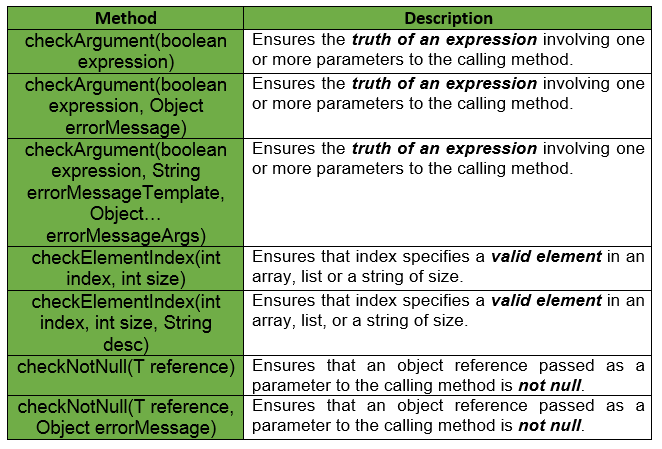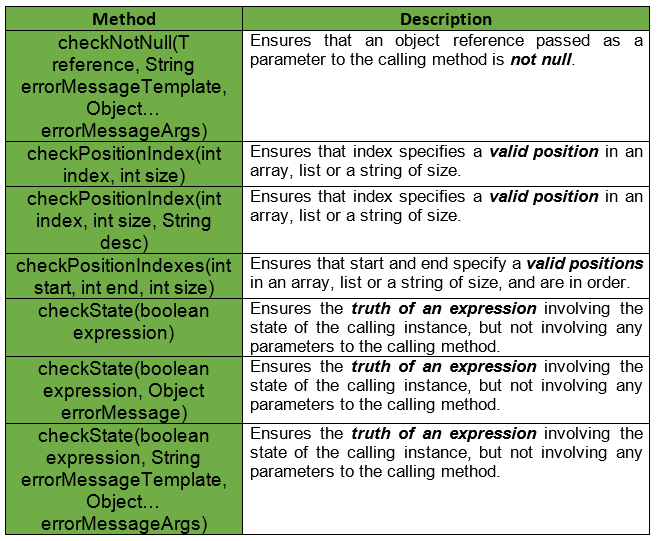Open in App
Not now

# Preconditions | Guava | Java

• Difficulty Level : Basic
• Last Updated : 18 May, 2018

Introduction : The Preconditions Class provides a list of static methods for checking that a method or a constructor is invoked with valid parameter values. If a precondition fails, a tailored exception is thrown.

• These methods generally accept a boolean expression which is expected to be true.
• In the case of checkNotNull, these methods accept object reference which is expected to be non-null.
• When false/null is passed instead, the Preconditions method throws an unchecked exception.

Declaration :

```@GwtCompatible
public final class Preconditions
extends Object
```

Example 1 : In this example, checkArgument throws an IllegalArgumentException to indicate that examplePre1 made an error in its call to sqrt as the passed argument is negative.

 `// Returns the positive square``// root of the given value.``// throws IllegalArgumentException``// if the value is negative``public` `static` `double` `sqrt(``double` `value)``{``    ``Preconditions.checkArgument(value >= ``0.0``, ``"negative value: %s"``, value);``    ``// calculate the square root``}`` ` `void` `examplePre1()``{``    ``// Function calling``    ``double` `ans = sqrt(-``5.0``);``}`

Example 2 : Let us say we are given a method which takes a List as an argument. When this method is invoked we want to check that the list is not null and it is not empty. A simple Java solution for the problem would look like :

 `// Method to check if passed list``// is null or empty``public` `void` `example(List

But when we use Guava’s Preconditions, the amount of code is reduced significantly. The solution looks something like :

 `// Method to check if passed list``// is null or empty``public` `void` `example(List

Example 3 : If one wants to validate age to make sure it is greater than 18, one can use Precondition.checkArgument().

 `// To check if age is greater than 18 or not``public` `static` `void` `validateAge(``int` `age)``{``    ``// Guava Preconditions``    ``checkArgument(age > ``18``);``}`

Following table shows some of the methods provided by Guava Preconditions.Each method has three variants, which are as listed below :

• No extra arguments : Any exceptions are thrown without error messages.
• An extra Object argument : Any exception is thrown with the error message object.toString().
• An extra String argument : This method takes an extra String argument with an arbitrary number of additional Object arguments. This behaves something like printf, but for GWT compatibility and efficiency, it only allows %s indicators.

Examples of the third variant :

```checkArgument(i >= 0, "Argument was %s but expected nonnegative", i);
checkArgument(i < j, "Expected i = %s", i, j);
```

Some other methods provided by Guava Preconditions are :Important Points : Below given are some important points regarding Guava Preconditions.

• It is of course possible to use the methods of this class to check for invalid conditions which are not the caller’s fault. Doing so is not recommended because it is misleading to future readers of the code.
• Projects which use com.google.common should generally avoid the use of Objects.requireNonNull(Object). Instead, use whichever of checkNotNull(Object) or Verify.verifyNotNull(Object) is appropriate to the situation.
• In Preconditions error message template strings, only the “%s” specifier is supported, not the full range of Formatter specifiers.

Example :

 `// Java code to show implementation of``// Guava Preconditions``import` `com.google.common.base.Preconditions;`` ` `class` `GFG {`` ` `    ``// Driver code``    ``public` `static` `void` `main(String args[])``    ``{`` ` `        ``// Creating object of GFG Class``        ``GFG obj = ``new` `GFG();`` ` `        ``// try block``        ``try` `{``            ``System.out.println(obj.sum(``5``, ``null``));``        ``}`` ` `        ``// catch block``        ``catch` `(NullPointerException e) {``            ``System.out.println(e.getMessage());``        ``}``    ``}`` ` `    ``// Method to compute sum of 2 Integers``    ``public` `int` `sum(Integer num1, Integer num2)``    ``{``        ``// Guava Preconditions``        ``num1 = Preconditions.checkNotNull(num1,``                                          ``"Illegal Argument, First parameter is Null."``);`` ` `        ``// Guava Preconditions``        ``num2 = Preconditions.checkNotNull(num2,``                                          ``"Illegal Argument, Second parameter is Null."``);`` ` `        ``return` `(num1 + num2);``    ``}``}`

Output :

```Illegal Argument, Second parameter is Null.
```